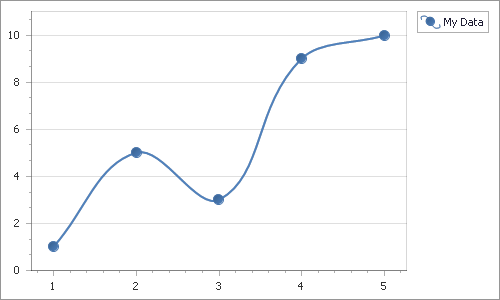# Overview

Chart is a visual extension used to display assorted data in a graphical representation. It visualizes the Series of Points, using one of the available View Types. Note that a chart can draw multiple series using different View Types at the same time.

## Implementation Details

Chart is represented by the ChartControlExtension class. Its instance can be accessed via the ExtensionsFactory.Chart helper method, which is used to add a Chart extension to a view. This method’s parameter provides access to the Chart‘s settings implemented by the ChartControlSettings class, allowing you to fully customize the extension.

Chart‘s client counterpart is represented by the MVCxClientChart object.

## Declaration

Chart can be added to a view in the following manner.

``````namespace MyProject.Models {
public class ChartPoint {
public int X { get; set; }
public int Y { get; set; }
}
}
``````
``````using System.Collections.Generic;
using System.Web.Mvc;
using MyProject.Models;

namespace MyProject.Controllers {
public class HomeController : Controller {
public ActionResult Index() {
return View(GetPoints());
}

private List<ChartPoint> GetPoints() {
var m = new List<ChartPoint>();
m.Add(new ChartPoint { X = 1, Y = 1 });
m.Add(new ChartPoint { X = 2, Y = 5 });
m.Add(new ChartPoint { X = 3, Y = 3 });
m.Add(new ChartPoint { X = 4, Y = 9 });
m.Add(new ChartPoint { X = 5, Y = 10 });
return m;
}
}
}
``````
``````@Html.DevExpress().Chart(settings => {
settings.Name = "chart";